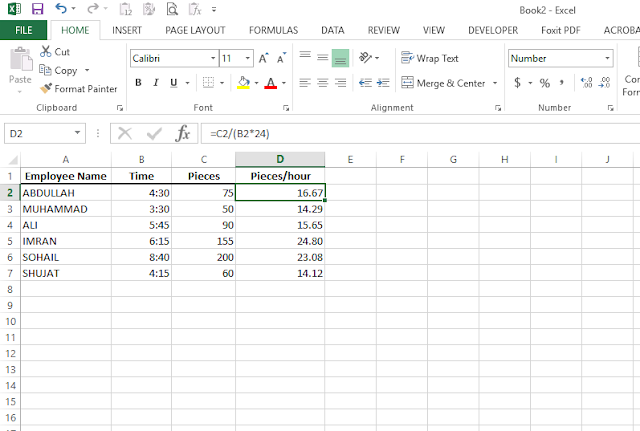# How To Measure Production Efficiency in excel

## How To Measure Production Efficiency in excel

### To Find Employee Of The Month in excel

In this post, we will show you How To Measure Production Efficiency, To Find Employee Of The Month, you can calculate the hourly output of an employee of any company with the help of Microsoft Excel.
As ever if you want to know who is the best employee of the month, you can do it with this formula or find out productive employees.
All this with a simple Microsoft Excel formula All just by the formula of division and multiplication

1. First, open Microsoft Excel Sheet
2. Then enter the names of the employees in column A.
3. Then in column B, write down the number of hours an employee worked.
4. Then in column C write down how much output each employee has.
5. Now put the Microsoft Excel formula =C2/(B2*24) in the D column.

After that, you'll have the answers to all the questions below.

What is the production efficiency formula?
How to calculate production efficiency in excel?
What is the net production efficiency formula?
How to find production plant efficiency calculation?
What is production line efficiency calculation?
What is the production efficiency formula in excel?
Production efficiency calculation formula?
Does production efficiency calculation excel?